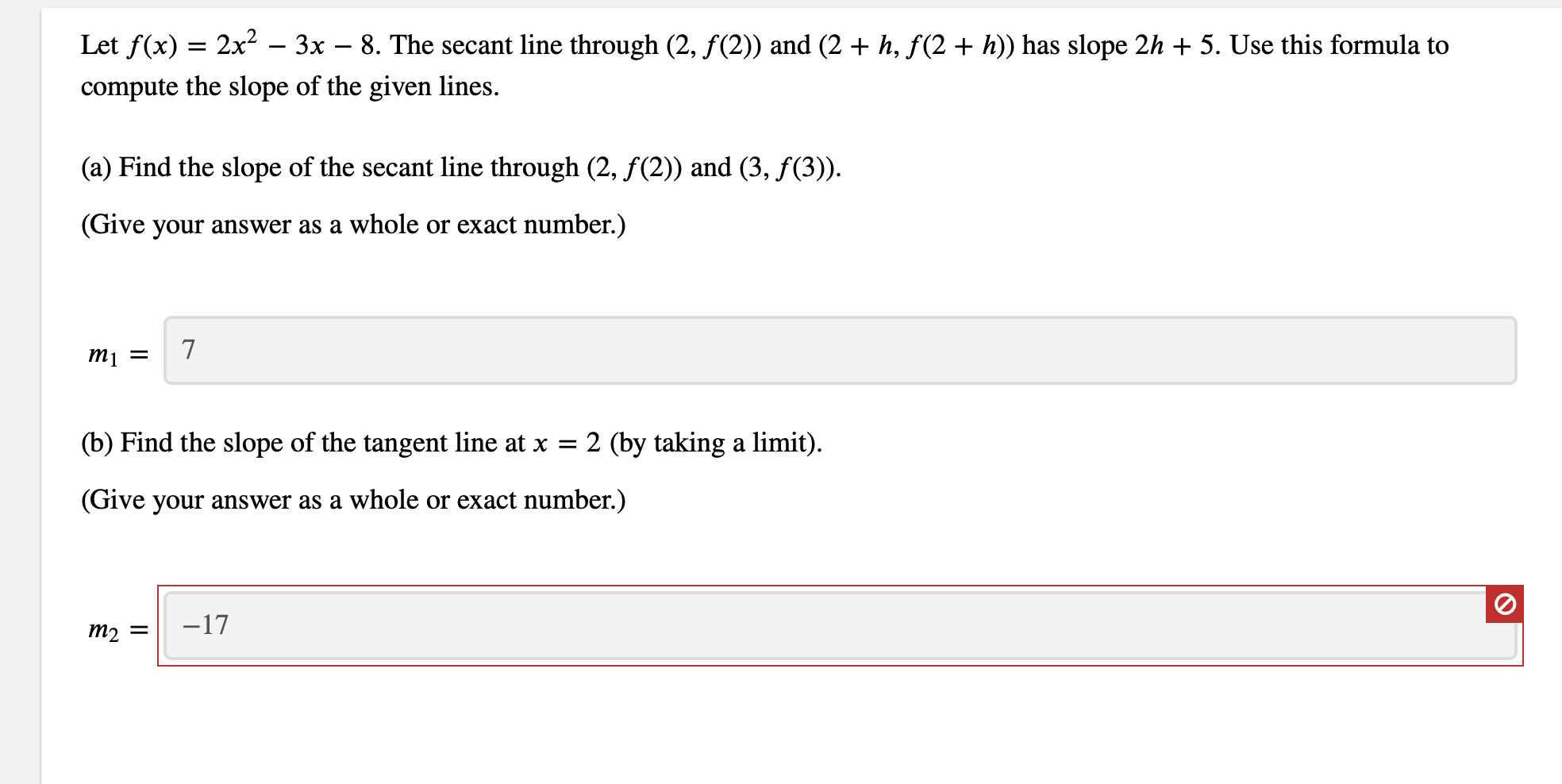Let f(x) 2x2 - 3x - 8. The secant line through (2, f(2)) and (2h, f(2 + h)) has slope 2h + 5. Use this formula tocompute the slope of the given lines(a) Find the slope of the secant line through (2, f(2)) and (3, f(3).(Give your answer as a whole or exact number.)7mj =2 (by taking a limit)(b) Find the slope of the tangent line at x(Give your answer as a whole or exact number.)-17т2

Questionhelp_outlineImage TranscriptioncloseLet f(x) 2x2 - 3x - 8. The secant line through (2, f(2)) and (2 h, f(2 + h)) has slope 2h + 5. Use this formula to compute the slope of the given lines (a) Find the slope of the secant line through (2, f(2)) and (3, f(3). (Give your answer as a whole or exact number.) 7 mj = 2 (by taking a limit) (b) Find the slope of the tangent line at x (Give your answer as a whole or exact number.) -17 т2 fullscreen
Step 1

(2+h,f(2+h))= (3,f(3))

This means 2+h=3

So, h=3-2=1

So slope of the secant line through (2,...

Want to see the full answer?

See Solution

Want to see this answer and more?

Our solutions are written by experts, many with advanced degrees, and available 24/7

See Solution
Tagged in

Calculus